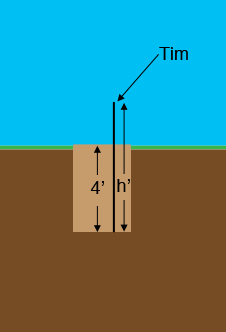SEARCH HOMEMath Central Quandaries & QueriesQuestion from Marvel, a parent: Tim is standing in a hole that is 4 feet deep. Ben asks him how much deeper is he going to dig the hole. Tim replies he will dig to the level that is 4 feet 2 inches deeper and that then the top of his head will be the same distance below ground level that it is now above. How tall is Tim?Hi Marvel,

I took Tim's height to be $h$ feet and drew a sketch of him in the 4 foot hole. Hence the top of Tim's head is $h - 4$ feet above the ground.Redraw the diagram with the hole 4 feet 2 inches deeper and the top of Tim's head $h - 4$ feet below the ground level. Use the diagram to write an equation for $h$ and then solve this equation.

Write back if you need more assistance,
PennyMath Central is supported by the University of Regina and The Pacific Institute for the Mathematical Sciences.